Next: 17.6 Substitution in Integrals Up: 17. Antidifferentiation Techniques Previous: 17.4 Integration by Substitution   Index

# 17.5 Trigonometric Substitution

Integrals of the form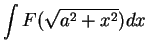and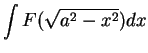often arise in applications. There is a special trick for dealing with such integrals. Since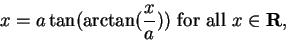we can write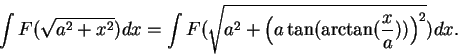If we now make the substitution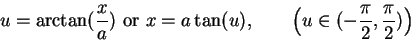then we find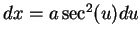, or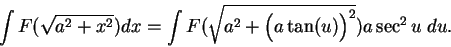Now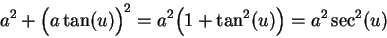so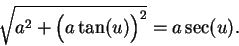(Since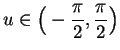we have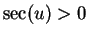and the square root is positive.) Thus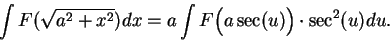Often this last antiderivative can be found. If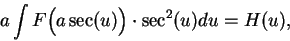then by the ritual (17.37)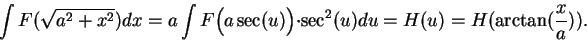The ritual to apply when using this method for findingis:

Let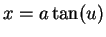. Then, and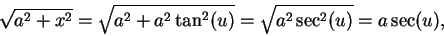so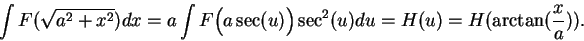There is a similar ritual for integrals of the form(Here we will just describe the ritual).

Let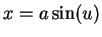. Then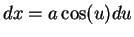and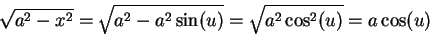(17.43)

so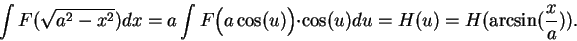Observe that in equation (17.43) we are assuming that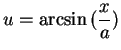, so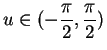, so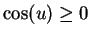, and the sign of the square root is correct.

17.44   Example. Find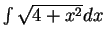.

Let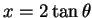. Then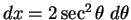, and(17.45)

Thus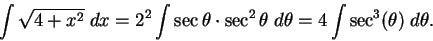To find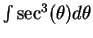, I will integrate by parts. Let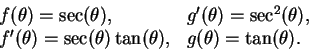Hence,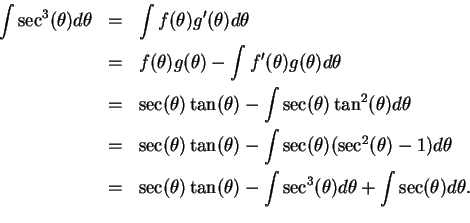Hence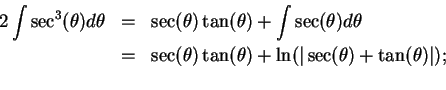i.e.,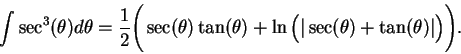(17.46)

Hence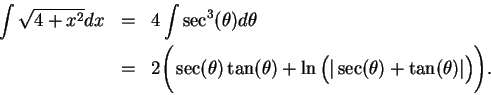By (17.45) we have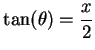and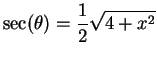. Thus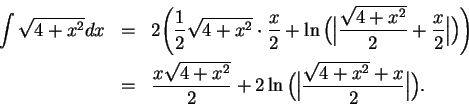17.47   Example. In the process of working out the last example we foundusing integration by parts. Here is a different tricky way of finding the same integral .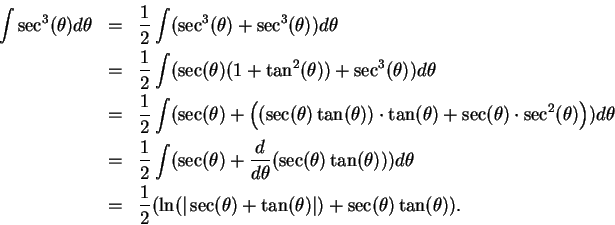17.48   Example. Find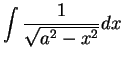.

Let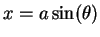. Then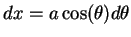and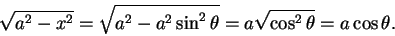Thus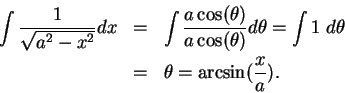17.49   Exercise. Find the following antiderivatives:
a)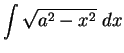b)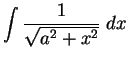c)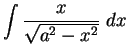d)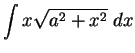A

17.50   Example (Area of a circular sector) Let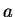be a positive number, and let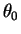be a number in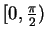. Let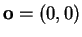, and let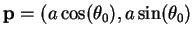. Let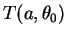be the circular sector bounded by the positive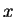-axis, the segment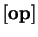, and the circle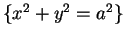.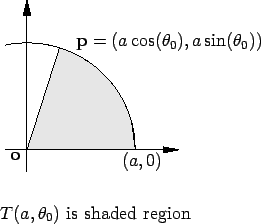The equation foris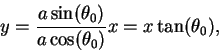and the equation for the upper semicircle is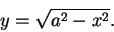Hence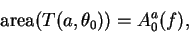where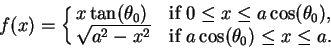i.e.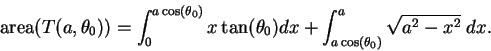In exercise 17.49A.a you showed that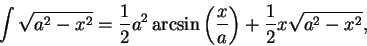so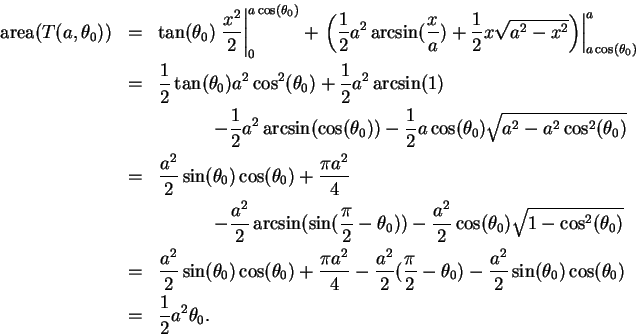By using symmetry arguments, you can show that this formula actually holds for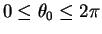.Next: 17.6 Substitution in Integrals Up: 17. Antidifferentiation Techniques Previous: 17.4 Integration by Substitution   Index
Ray Mayer 2007-09-07